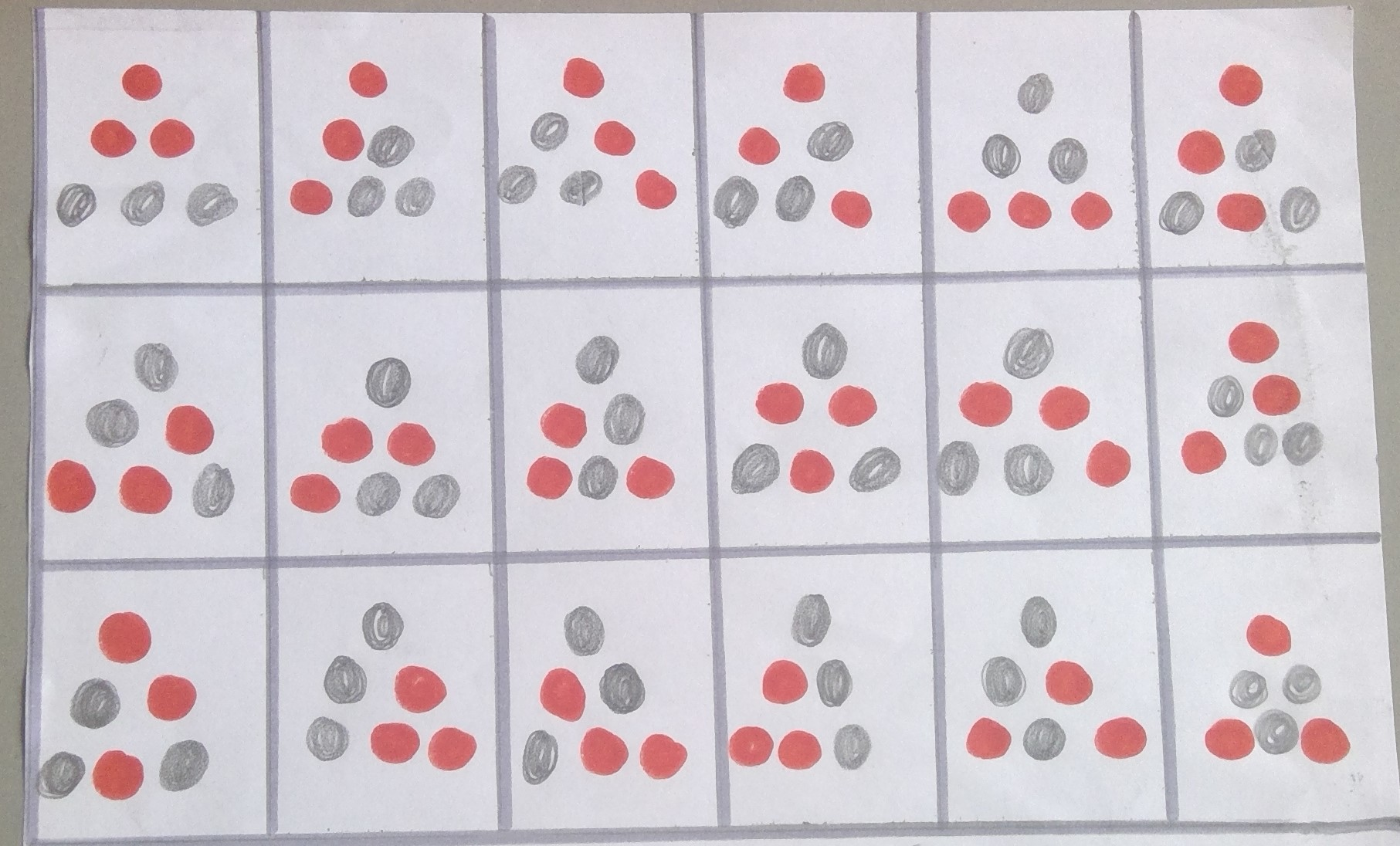#### You may also like### Stop or Dare

All you need for this game is a pack of cards. While you play the game, think about strategies that will increase your chances of winning.### Snail Trails

This is a game for two players. You will need some small-square grid paper, a die and two felt-tip pens or highlighters. Players take turns to roll the die, then move that number of squares in a straight line. Move only vertically (up/down) or horizontally (across), never diagonally. You can cross over the other player's trails. You can trace over the top of the other player's trails. You can cross over a single trail of your own, but can never cross a pair of your trails (side-by-side) or trace over your own trail. To win, you must roll the exact number needed to finish in the target square. You can never pass through the target square. The game ends when a player ends his/her trail in the target square, OR when a player cannot move without breaking any of the rules.### Game of PIG - Sixes

Can you beat Piggy in this simple dice game? Can you figure out Piggy's strategy, and is there a better one?

# Cosy Corner

##### Age 11 to 14Challenge Level

Ruby and Emma from Yewdale Primary in England used the interactivity to estimate the chance of winning:
For our working out we decided to look at how many wins we got out of 1000
100: 93     0.930
100: 92     0.920
100: 97     0.970
100: 95     0.950
100: 93     0.930
100: 97     0.970
100: 98     0.980
100: 93     0.930
100: 93     0.930
100: 95     0.950

Daniel from Bayside School in Gibraltar and Sanika P from PSBBMS, OMR in India used probability directly. This is Daniel's work:
There are 6 positions that a ball can take, and out of those 6, 3 are corners.

To lose, you must end up with no red ball in a corner, and for that to happen, the 3 non corner spaces must each be taken up by a red ball.

For 1 red ball to take up a non corner space, the probability is $\frac36$

Given that one red ball is in a non corner position, the probability that the next red ball ends up in a non corner position is $\frac25$, and given that the two red balls are in central positions, the probability that the next red ball does not end up in a corner is $\frac14$.

The probability of no red ball landing in a corner spot is $\frac36\times\frac25\times\frac14$ which is $\frac1{20}$.

So the probability that a red ball does land in a corner is $1-\frac1{20}=\frac{19}{20}=0.95$

Layla and Tamara from Frederick Irwin Anglican School in Australia counted the number of winning arrangements:
You want to start by deciding and experimenting with however many different possibilities there is for the red balls to settle in. To help with this we tried mirroring and reversing patterns to make more. We came up with the answer of 18 different positions. You must then figure out how many of those positions can no red balls be in the corners. The answer to that is only one position! So the formula would be approximately 1 in 18 tries that you would lose the game.Layla and Tamara got 1 in 18 instead of 1 in 20 beacuse there are two arrangements missing:Using a systematic counting method like the one below can help us be sure there aren't any more missing:
red in 0 corners1 arrangement

red in 1 corner3 options for red corner, 3 options for which ? is not red:
9 arrangements

red in 2 corners3 options for not red corner, 3 options for which ? is red:
9 arrangements

red in 3 corners1 arrangement

Jacob from Acland Burghley in the UK used factorials:
I already know that to work out the number of ways of arranging a number of colours or in this case counters is the factorial of that number.

The factorial of $4$ is written $4!$ and means $4\times3\times2\times1$. There are $4!$ ways to arrange $4$ counters in $4$ spaces because:
There are $4$ empty spaces in which to place the first counter
For each of these $4$ options for the first counter, there will be $3$ options left for the second counter
For each of these $4\times3$ arrangements for the first two counters, there will be $2$ options left for the third counter, and only $1$ for the fourth.

The red tiles aren't interchangeable because when it is shuffling they are kept independent. With these two facts I know there are $720$ arrangements ($6!$).

The number of arrangements where you lose is $36$ because there are $6$ ways of arranging the $3$ reds that all need to be in the middle, and $6$ ways of arranging the outer counters in each way of arranging the middle ones, so $6\times6$ is how many ways to lose there are.

$720\div36=20$ so you lose $1$ in $20$.